Modeling

We developed a mathematical model for Korteweg stresses in miscible fluids in which the stress tensor terms are added to the incompressible Navier-Stokes equation. We consider mass, energy and momentum conservation. We assume:
a) Thermal and diffusive fluxes are small,
b) The internal energy has the form,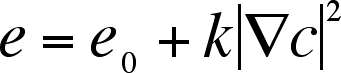c) Capillary forces are tangential to the composition gradient,
d) The fluids are incompressible and have the same density and viscosity.
We obtain the following model.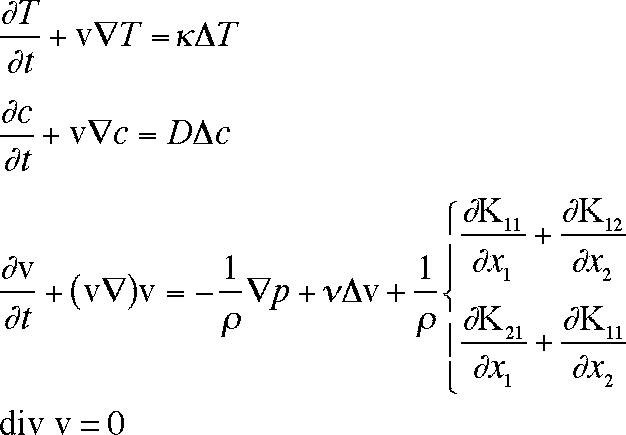Here T is the temperature, v is the fluid velocity, p is the pressure, n is the kinematic viscosity, r is the density, and c is the concentration (mole fraction) of polymer. Kij are the stress tensor terms, defined by: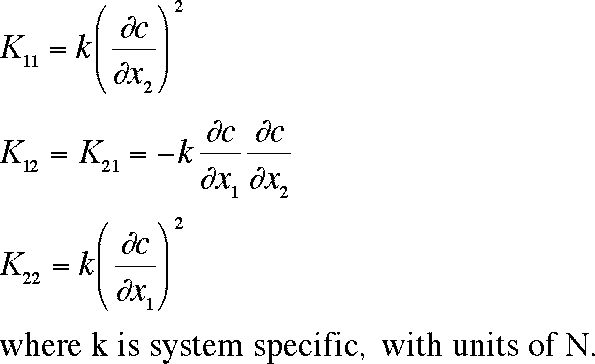In this analysis we use an adaptive grid formulation. Our method employs conservation laws (mass, momentum, energy) in integral form. Using integral forms instead of differentials enables the use of a viscosity that is dependent on concentration and temperature. In addition we use a “pressure-velocity” instead of the “stream-function and vorticity” as our basic variables.

For the initial width of the transition zone we used a value of 0.1 mm. The square gradient parameter, k, was varied until we reached a value at which no change in the drop shape was observed.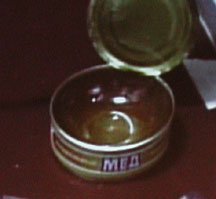We obtained two cans of the same type of honey used on the ISS and measured its viscosity as a function of honey concentration and temperature (Fig. 11) using an Anton Paar rheometer. From these data we generated a function where C=1 for pure honey and C = 0 for pure water.

Viscosity (c,T) = (41.5 x 10–5– 1.07 x 10–5T) e-0.1 C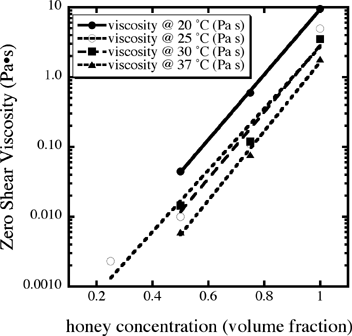Fig. 11 The zero-shear viscosity as a function of honey fraction in water and temperature.

Diffusion Coefficient as a function of concentration and temperature
We used the data for fructose, the largest component of honey, from Sano et al.  for the diffusion coefficient as a function of temperature and concentration:

d (c, T) = 7 x 10–10 x 10–a (T) C , kg/(m s)
a(T) = 4.81 + 8.42/(T+273)

For the simulation of the water injected into honey, we used the image to define the initial conditions. For the stream of honey injected, we only simulated the tip. If the tip does not exhibit any change in shape where the curvature is greatest, then we do not expect the stream to break up.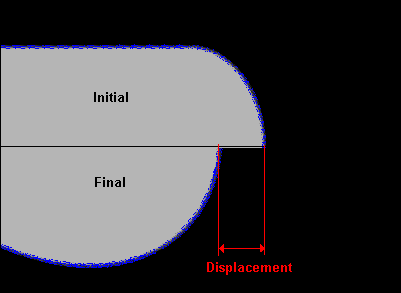Fig. 12 The initial drop shape for the simulation of a blob of water injected into honey was taken from the experiments. The displacement would be negative for this case. d = 0.1 mm

Water into honey
Fig. 12 shows the initial conditions and how the drop can change shape because of the Korteweg stress. By plotting the displacement of the tip we can determine if any significant fluid motion should occur for different values of the square gradient parameter. We note first that the tip initially stretches out and then the pulls back. Fig. 13 compares two simulations, one with k = 10–10 N and k = 10–12 N. In both cases the drop makes a small positive displacement (to the right) and then contracts. Fig. 14 compares both cases with two more in which the concentration of honey is decreased. Because the viscosity is a strong function of concentration, the amount of displacement is much more pronounced.
In all cases, a very small displacement would be observed after 10 minutes (600 seconds). We had only requested the observation to proceed for 10 minutes but for the last run by John Phillips, we requested an extension to 30 minutes using the digital camera’s intervalometer. However, bubbles were drawn into the system, eliminating the value of the run.

Fig. 13. The evolution of a water drop in pure honey for 10–11 N (Left) and k = 10–12 N (Right)

The height of the domain shown is 0.7 cm, which is half of the total simulation domain.

Fig. 14. Isothermal simulation results for water into honey with initial conditions shown in Fig. 12. Results for water into pure honey and water into 80% honey. Notice the significant effect of a 20% change in honey concentration. 10–11 N (Left) and k = 10–12 N (Right)

Using the data from Mike Fincke, we calculate the temperature gradient of 5 K over 1.9 cm. We assume it is linear and does not change during the simulations. Our model is axisymmetric, so during its motion the drop conserves its axisymmetric shape. However, the temperature gradient can still affect the evolution of the drop by creating gradients of the transition zone width. With k = 10–11 N, significant change in the tip shape can be observed (Fig. 15). In Fig. 16, the tip shape also changes significantly. For k = 10–12 N, some change in the tip shape might be observable. Since we did not observe such behavior, we can estimate that k <10–12 N.

Fig. 15 The evolution of the tip of in pure honey injected into water with an applied temperature gradient, for k = 10–11 N (Left) and k = 10–12 N (Right). The temperature gradient was 2 K cm–1.

Discussion

No movement of the drops or streams was observed that could not have been caused by residual acceleration in the ISS. If our Korteweg-stress model is correct and if our functions for the viscosity and diffusion coefficient dependences on temperature and concentration are valid, then we can estimate that the square gradient parameter for honey and water is less than 10–12 N.
Although these experiments are the first of their kind, they are relatively crude and filled with uncertainty. The concentrations of water and honey were only estimated by the crewmember. The properties of the honey used in the experiments can only be estimated from the sample we obtained. Finally, we used data for fructose’s diffusion coefficient but honey is a supersaturated solution containing many compounds.

Conclusions
For the first time, miscible fluids were studied in microgravity to determine if they could exhibit transient interfacial phenomena seen with immiscible fluids. Four experiments were performed with honey and water under isothermal conditions. For a stream of honey injected into water, no Rayleigh-Tomotika instability was observed. A blob of water did not appear to change its shape. Numerical simulations indicate that if the Korteweg-stress model is correct then the square gradient parameter must have a value < 10–12 N.
Two experiments were performed with temperature gradients. For the injection of diluted honey into water, the stream migrated to the high temperature side of the syringe but the injection process caused this. Simulations with a temperature-independent square gradient parameter but a temperature-dependent diffusion coefficient revealed that the a measurable change in the tip of the drop would have been observed if k ≥ 10–12 N.
With all the limitations of a zero-upmass investigation, MFMG was a success and will hopefully motivate additional microgravity studies with miscible fluids.

ACKNOWLEDGMENTS

Support for this project was provided by NASA’s Microgravity Materials Science Program (NAG8-1466). We thank the MFMG team for their hard work and perseverance: Melanie Bodiford, Larry Cotton, Glen Funkhouser, Lucinda Murphy, Julia Ogle, Frank Parris, John Yates and Ron Young. We also are deeply indebted to Dr. Michael Foale, Mike Fincke, Leroy Chiao and Dr. John Phillips for their skill and hard work. We also thank Joshua Otaigbe and Kevin Urman for measurement of the viscosity and thank Anton Paar Physica for the donation of the rheometer.

References

Back to the Main MFMG Page